# Problem 55335. Generate Bernoulli polynomials

The Bernoulli polynomial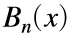is a polynomial of order n with the properties that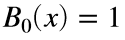and for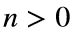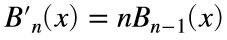,
where the prime denotes differentiation with respect to x, and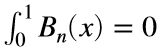.
Therefore,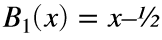,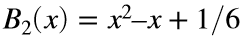,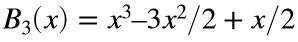, etc. Notice that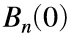is the nth Bernoulli number.
Write a function to generate the Bernoulli polynomial of order n. Use MATLAB's approach for specifying the coefficients. For example, the function should return [1 -1/2] for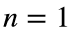and [1 -1 1/6] for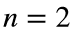.

### Solution Stats

58.33% Correct | 41.67% Incorrect
Last Solution submitted on Sep 15, 2023

### Community Treasure Hunt

Find the treasures in MATLAB Central and discover how the community can help you!

Start Hunting!